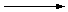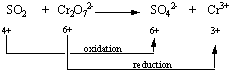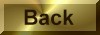Practice Problem 7

Write a balanced equation for the disproportionation of bromine in the presence of a strong base.

Solution

STEP 1: Write a skeleton equation for the reaction.

Br2 + OH-Br- + BrO3-

STEP 2: Assign oxidation numbers to atoms on both sides of the equation.
 Br2 + OH-Br - + BrO3- 0 -2 +1 -1 +5 -2

STEP 3: Determine which atoms are oxidized and which are reduced.STEP 4: Divide the reaction into oxidation and reduction half-reactions and balance these half-reactions. Bromine is oxidized from the 0 to the +5 oxidation state in this reaction.

 Oxidation: Br2BrO3- 0 +5

Bromine is also reduced in this reaction, from the 0 to the -1 oxidation state.

 Reduction: Br2Br- 0 -1

If we assume that two Br- ions are produced for each molecule of Br2 reduced, the reduction half-reaction would be written as follows.

 Reduction: Br2 + 2 e-2 Br -

If we assume that two BrO3- ions are formed each time a Br2 molecule is oxidized, the oxidation half-reaction involves the loss of 10 electrons.

 Oxidation: Br22 BrO3- + 10 e-

Because the reaction occurs in basic solution we can add OH- ions or H2O molecules to either side of the equation, as needed. The trick, as always, is deciding which side of the equation gets the OH- ions and which side gets the H2O molecules. In this case, the only way to balance the charge on both sides of the equation is to add 12 OH- ions to the reactants.

 Oxidation: Br2 + 12 OH-2 BrO3- + 10 e-

Six of the oxygen atoms in the OH- ions end up in the BrO3- ions. What happens to the other six oxygen atoms and the 12 hydrogen atoms? The most reasonable answer is that they combine to form six H2O molecules.

 Oxidation: Br2 + 12 OH-2 BrO3- + 10 e- + 6 H2O

STEP 5: Combine the two half-reactions so that electrons are neither created nor destroyed. Ten electrons are given off in the oxidation half-reaction and two electrons are consumed in the reduction half-reaction. We can therefore combine these half-reactions as follows.

 5(Br2 + 2 e-2 Br-) + (Br2 + 12 OH-2 BrO3- + 10 e- + 6 H2O) ___________________________________________ 6 Br2 + 12 OH-10 Br- + 2 BrO3- + 6 H2O

STEP 6: Balance the remainder of the equation by inspection, if necessary. The equation generated in the previous step is balanced, but it is not quite correct. The best answer to this exercise would be the equation with the smallest possible coefficients, so we divide all of the coefficients by 2.

3 Br2 + 6 OH-5 Br- + BrO3- + 3 H2O

We now complete the exercise by identifying the states of the reactants and products, as follows.

3 Br2(aq) + 6 OH-(aq)5 Br-(aq) + BrO3-(aq) + 3 H2O(l)